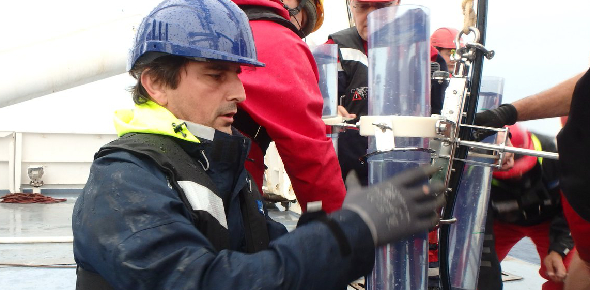# Quiz 9: Hydrostatics

10 Questions | Total Attempts: 232SettingsYou may take this quiz up to 3 times; I will keep your highest score. The quiz is open through Sunday, 11/20.

• 1.
If the distance between two objects is doubled, what happens to the force of gravitational attraction between them?
• A.

It stays the same

• B.

It is cut in half

• C.

It doubles

• D.

It is 1/4 as strong

• 2.
When calculating the force of gravity between two objects, you need to measure the distance between:
• A.

The centers of mass of the two objects

• B.

The points where the objects surfaces are closest to each other

• C.

The points where the objects surfaces are farthest away from each other

• D.

Two arbitrary points within the objects' mass

• 3.
According to Keler, the path of a planet's orbit is in the shape of _____________.
• A.

A circle, with the sun at the center

• B.

An ellipse, with the sun at one of the foci

• C.

A circle, with the earth at the center

• D.

An ellipse, with the earth at one of the foci

• 4.
When playing billiards, the collisions between the balls are best described as __________________.
• A.

Elastic

• B.

Inelastic

• C.

Expensive

• D.

Exhaustive

• 5.
The kinetic energy of an object will increase the most if you __________________.
• A.

Double the velocity of the object

• B.

Double the mass of the object

• C.

Double the potential energy

• D.

Double the temperature of the object

• 6.
Atmospheric pressure at sea level is caused by ________________.
• A.

The force of gravity on the air

• B.

The potential energy of molecules

• C.

The gravitational pull of the sun

• D.

The vacuum of space

• 7.
Atmospheric pressure is pushing in on us all the time, but we don't feel it because ___________________.
• A.

It only works on gas

• B.

It is balanced by the pressure of the fluid in our cells

• C.

Gravity cancels it out

• D.

We can't feel air

• 8.
Water pressure increases the farther down you go because ________________________.
• A.

You are closer to the center of the earth

• B.

The water molecules are packed together more tightly

• C.

The weight of all of the water above you increases the pressure

• D.

You get smaller the farther down you go

• 9.
An object will float in a fluid if ______________________.
• A.

The fluid displaced by the object weighs more than the object

• B.

The object is small

• C.

The fluid is colder than the object

• D.

The fluid is a liquid, not a gas

• 10.
If you have a helium balloon floating behind you in your car, and you turn sharply to the left, the balloon will ______________.
• A.

Move to the right of the car because of inertia keeping it moving in a straight line

• B.

Be forced to the left of the car by the inertia of the air in the car displacing it

• C.

Not move because it is lighter than the air around it

• D.

Pop

Related Topics